Open in App
Not now

# How to Add Mixed Fractions with Different Denominators?

• Last Updated : 29 Jan, 2022

Fractions can be defined as numbers which can be represented in the form of A/B where A and B are integers and B should not be equal to zero. In a fraction, the upper part is called Numerator and the lower part is called the Denominator.

Examples: 1/2, 4/5, -2/3 etc.

### Mixed Fractions

Mixed Fractions can be defined as fractions that contain two parts one is called the Whole part and the other is called the fraction part.

Example: 1×(1/2) in which 1 is the whole number part and the other is the fractional part which is 1/2.

To add mixed fractions, first convert the mixed fraction to simple fractions by using the below method:

Given 5×(2/3) which is a mixed fraction, now to convert it to a simple fraction we need a numerator and a denominator.

Formula for finding numerator

Multiply the whole number part with the denominator of the mixed part and add a numerator of the mixed part.

So numerator = 5×3 + 2 = 17

Denominator will be same so denominator = 3

Hence mixed fraction is converted to simple fraction 5×(2/3) = 17/3

Now add fractions by the following the procedure :

To add fractions there is a rule which states that denominators of the fractions to be added should be equal. If the denominators of the fraction are not equal then make them equal by taking the Lowest Common Multiple(LCM) of the denominators.

How to find LCM?

To find the LCM of numbers (here denominators), we will use Division Method.

Let’s understand this method with the help of an example, take two numbers 4 and 15 for finding LCM using the division method.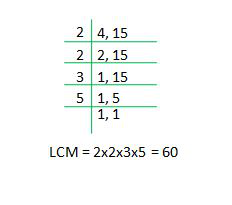Step 1: Make a table that contains a left-hand side and a right-hand side, on the right-hand side put numbers whose LCM we are finding.

Step 2: Now start with the smallest number (not 1) and check if any number from the given numbers has this as its multiple. In the example, 2 is the factor of 4 so use it to divide 4 in the next row.

Step 3: Now in second row 2, 15 are left now, only factor of 2 is 2 so take 2 to divide it.

Step 4: Now we have 1, 15 in the next row so 3 is the factor of 15 so divide 15, the result is 1, 5.

Step 5: Now 5 is the factor of 5 so divide 5, the result is 1, 1.

Step 6: The process is complete as we get 1 for all numbers, now multiply all the numbers on the left-hand side which are 2,2,3,5 so multiple of these is 60.

### Addition of mixed fractions with different denominators

Steps to add mixed fractions with different denominators are:

Step 1: Convert mixed fractions to simple fractions.

Step 2: Find LCM of denominators.

Step 3: Divide the LCM by the denominator of each number which are to be added.

Step 4: Multiply the numerator with the quotient ( found in the above step).

Step 5: Add the numerators we get after multiplying with quotients like simple addition.

Step 6: The denominator will be the LCM.

Example

Let’s take 2 mixed fractions with different denominators, 1×(1/3), 4×(5/8).

Step 1: Converting mixed fractions to simple fractions by multiplying whole part with denominator and adding the numerator, to get the numerator of the simple fraction and denominator will be same.

So 1×(1/3) = 4/3

and 4×(5/8) = 37/8

Step 2: Finding LCM of 3 and 8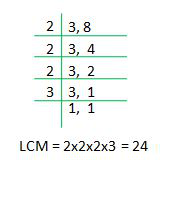Step 3: Divide the LCM by the denominator of each number which are to be added.

LCM = 24 so divide it by each number (denominator)

24/3 = 8 it is quotient 1

24/8 = 3 it is quotient 2

Step 4: Multiply the numerator with the quotient (found in the above step).

Numerators are 4 and 37 so multiply these with respective quotients.

4×8 = 32

37×3 = 111

Step 5: Add the numerators we get after multiplying with quotients like simple addition.

32 + 111 = 143 which is the numerator.

Step 6: Denominator will be the LCM so it is 24.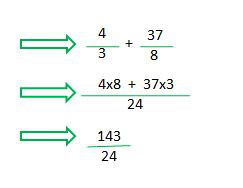### Cross Multiplication Method

Taking the example 1/2, 2/3

Step 1: Take two fractions for addition 1/2 and 2/3

Step 2: First we will find the numerator terms so we multiply the numerator of the first number with the denominator of the second number and similarly we will multiply the numerator of the second number with the denominator of the first number and add both the terms to get numerator.

1×3 + 2×2 = 7 which is numerator

Step 3: Now let’s find the denominator, for this multiply the denominator of the first term with the denominator of the second term to get the denominator term.

2×3 = 6 which is the denominator.

Step 4: We find the new term which is the addition of two fractions in this case new fraction is 7/6.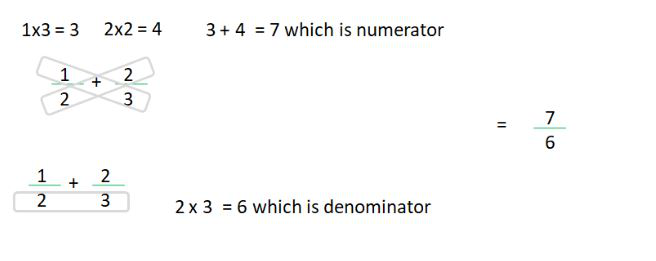### Sample Questions

Question 1: Add the given mixed fractions, 2×(1/7) and 3×(1/7).

Convert these fractions to simple fractions so 2×(1/7) = 15/7 and 3×(1/7) = 22/7.

In the given question the denominators are equal so simply add the numerators and the denominator will be 7.

Adding numerators 15 + 22  = 37

Denominator = 7

Question 2: Find the LCM of 4, 7, 12.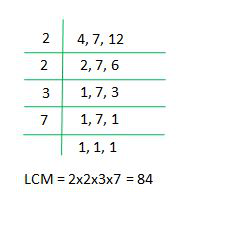Question 3: Add the given mixed fractions, 1×(1/2), 1×(1/3) and 1×(1/5).

Step 1: Convert the mixed fractions to simple fractions so 1×(1/2) = 3/2

1×(1/3) = 4/3  and 1×(1/5) = 6/5.

so fractions are 3/2,  4/3 and 6/5.

Step 2: Finding LCM of 2,3,5

LCM we got is 30.

Step 3: Divide the LCM by the denominator of each number which are to be added.

LCM = 30 so divide it by each number (denominator)

30/2 = 15 it is quotient 1

30/3 = 10 it is quotient 2

30/5 = 6 it is quotient 3

Step 4: Multiply the numerator with the quotient ( found in the above step).

Numerator are 3, 4, 6 so multiply these with respective quotients.

3×15 = 45

4×10 = 40

6×6 = 36

Step 5: Add the numerators we get after multiplying with quotients like simple addition.

45 + 40 + 36 = 121 which is the numerator.

Step 6: Denominator will be the LCM so it is 30.

Question 4: Add the given mixed fractions, 1×(2/13), 2×(1/2) by cross multiplication method.

Step 1: Converting mixed fractions to simple fractions so

1×(2/13) = 15/13

2×(1/2) = 5/2

Step 2: Taking  15/13 and 5/2

Step 3: First we will find the numerator terms so we multiply the numerator of the first number with the denominator of the second number and similarly we will multiply the numerator of the second number with the denominator of the first number and add both the terms to get the numerator.

15×2 + 13×5 = 95 which is numerator

Step 4: Now let’s find the denominator, for this multiply the denominator of the first term with the denominator of the second term to get the denominator term.

13×2 = 26 which is the denominator.

Step 5: We find the new term which is the addition of two fractions in this case new fraction is 95/26.

Questions 5: Add 1×(1/3) and 4×(5/8) using cross multiplication.

Convert 1×(1/3) and 4×(5/8) to simple fractions so

1×(1/3) = 4/3

4×(5/8) = 37/8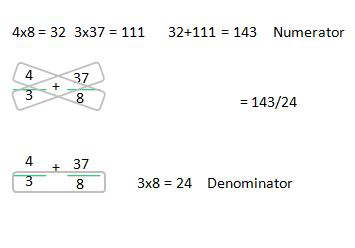My Personal Notes arrow_drop_up
Related Articles